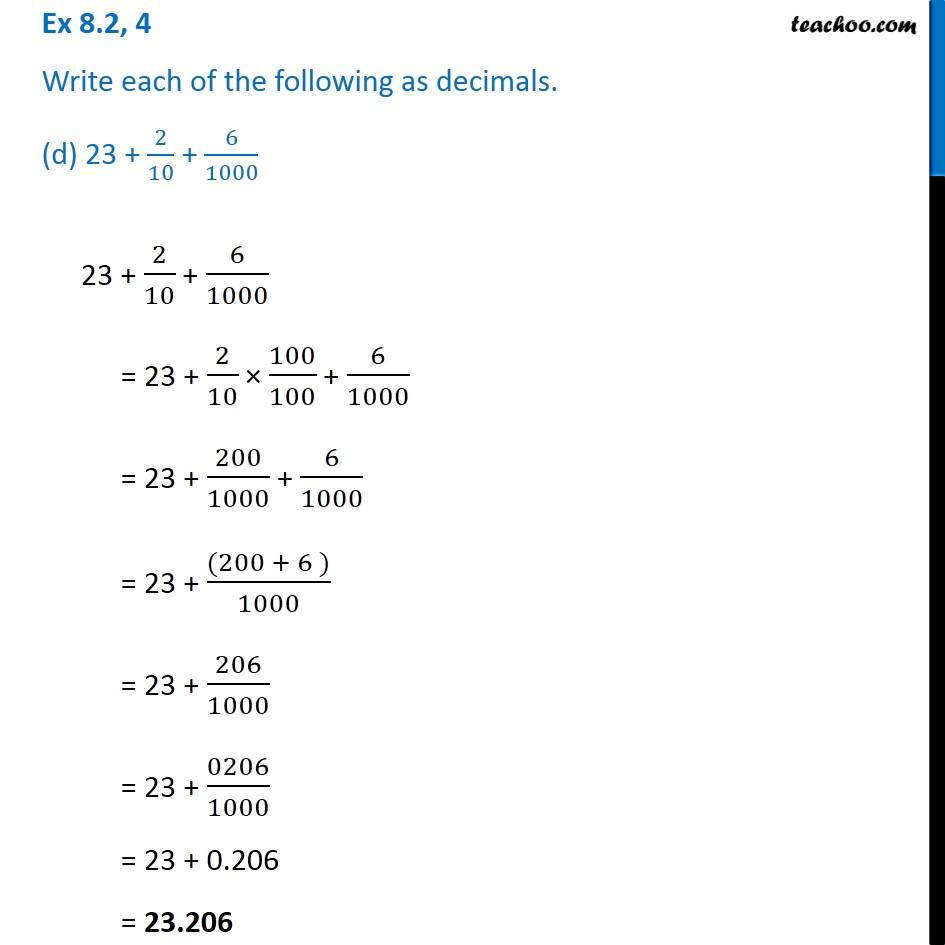Ex 8.2

Chapter 8 Class 6 Decimals
Serial order wiseGet live Maths 1-on-1 Classs - Class 6 to 12

### Transcript

Ex 8.2, 4 Write each of the following as decimals. (d) 23 + 2/10 + 6/1000 23 + 2/10 + 6/1000 = 23 + 2/10 100/100 + 6/1000 = 23 + 200/1000 + 6/1000 = 23 + ((200 + 6 ))/1000 = 23 + 206/1000 = 23 + 0206/1000 = 23 + 0.206 = 23.206